Courses

# Test: Shapes And Space - 2

## 10 Questions MCQ Test Mathematics (Maths) for Class 1 | Test: Shapes And Space - 2

Description
This mock test of Test: Shapes And Space - 2 for Class 1 helps you for every Class 1 entrance exam. This contains 10 Multiple Choice Questions for Class 1 Test: Shapes And Space - 2 (mcq) to study with solutions a complete question bank. The solved questions answers in this Test: Shapes And Space - 2 quiz give you a good mix of easy questions and tough questions. Class 1 students definitely take this Test: Shapes And Space - 2 exercise for a better result in the exam. You can find other Test: Shapes And Space - 2 extra questions, long questions & short questions for Class 1 on EduRev as well by searching above.
QUESTION: 1

Solution: A
QUESTION: 2

### What is the name of this shape?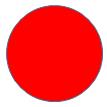Solution:

A completely symmetrical round shape is called circle.

QUESTION: 3

### Find the missing letters : T_IA_G_E

Solution: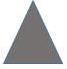QUESTION: 4

How many circles are shown below?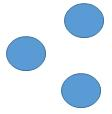Solution:
QUESTION: 5

Match the shape to its name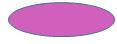Solution:
QUESTION: 6

Find the missing letters : C_B_

Solution:

Answer is CUBE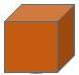QUESTION: 7

Find the Triangle

Solution:This is a Triangle.

QUESTION: 8

Match the shape to its name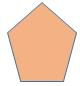Solution:

A figure having 5 sides is called pentagon.

QUESTION: 9

Square has _______ equal sides

Solution: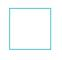Square has 4 equal sides.

QUESTION: 10

Find the Oval

Solution: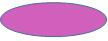This is Oval in shape.